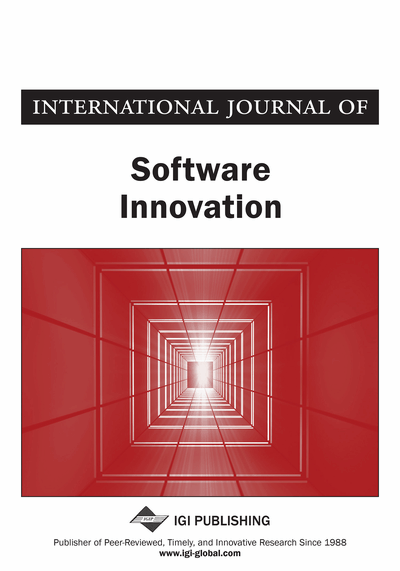# Recovering Polyp Shape from an Endoscope Image Using Two Light Sources

Hiroyasu Usami (Department of Computer Science, Chubu University, Kasugai, Japan), Yuji Iwahori (Department of Computer Science, Chubu University, Kasugai, Japan), Yuki Hanai (Department of Computer Science, Chubu University, Kasugai, Japan), Boonserm Kijsirikul (Department of Computer Engineering, Chulalongkorn University, Bangkok, Thailand) and Kunio Kasugai (Department of Gastroenterology, Aichi Medical University, Nagakute, Japan)
DOI: 10.4018/IJSI.2017040103

## Abstract

This paper proposes a new approach to recover the polyp shape from an endoscope image using a photometric constraint equation considering two light sources. The procedures are as follows. First, obtain the initial depth distributions by optimizing photometric equation obtained from two light sources. Next, obtain the surface normal vector from depth using numerical difference at each point. Then the mapping between the obtained normal vector and true normal vector is learned using Radial Basis Function Neural Network for a Lambertian sphere, and learning is generalized to another actual polyp image. Finally, optimize the depth using the obtained surface normal to recover the final 3D shape. The validity is confirmed of this method in comparison with the previous methods via computer simulation and experiments using actual endoscope images.
Article Preview
Top

## Introduction

Endoscope has been widely used in the medical field for polyp diagnosis. Those diagnoses depend on the experience of the medical doctor. It is hoped to obtain the detailed shape so that medical diagnosis will be more effective.

Specialized endoscopes with a laser light beam head (Nakatani, Abe, Miyakawa, & Terakawa, 2007) or with two cameras mounted in the head (Mourgues, Devemay, & Coste-Maniere, 2001) have been developed. The problem considered is the recovery of the 3-D shape of tissue in view. Stereo based endoscopy is one approach (Thormahlen, Broszio, & Meier, 2002). The challenge with stereo endoscopy is to determine corresponding features in the two images while the shape of internal organs itself is changing. Here, we consider a general purpose endoscope, of the sort still most widely used in medical practice.

As a method to recover shape from image, Shape from Shading (SFS) has been proposed. SFS was originally formulated by Horn (Horn & Brooks, 1989) to recover 3D shape from a 2D image. With a single camera endoscope, shape from shading can be applied. The Fast Marching Method (FMM) (Kimmel & Sethian, 2001) is a useful approach as an extension of SFS under the assumption of parallel light source and orthographic projection. Furthermore, as an extended approach to endoscope image based on SFS under the condition of point light source and perspective projection, a method (Iwahori et al., 2015) has been proposed to recover the polyp shape using both photometric and geometric constraints. The method assumes the condition of Lambertian reflectance and uses an objective function to find surface gradient parameters which minimizes the difference between the depth obtained from the photometric constraint equation and that obtained from the geometrical constraint equation. Once the gradient parameters at an interesting point, depth is calculated using the gradient parameters from the photometric constraint equation. However, the method obtains incorrect depth value for discontinuous surface since the method assumes a continuous smooth surface.

Another method (Tsuda et al., 2015) recovered shape of a target by solving the Hamilton-Jacobi equation which represents an equation as a wave of light particle using the Vogel-Breuβ-Weickert (VBW) model (Vogel, Breuβ, & Weickert, 2007). Equation is solved by combining Prados (Prados & Faugeras, 2003) and Faugeras (Prados & Faugeras, 2004) equations. However, the accuracy becomes low in these methods due to assuming one light source in case that actual endoscope has two light sources.

As a method of assuming multiple point light sources, a method (Wu, Narasimhan, & Jaramaz, 2010) has been proposed. The method tries to recover shape of spine under the assumption that the spine has Lambertian reflectance. However, if the recovery target is a polyp, since the polyp image includes blood vessels, diffuse reflection and specular reflection component, Lambertian processing is required. Therefore, this method cannot be applied to recover the polyp shape by itself. In addition, this method recovers the shape from multiple images using shape from shading and shape from motion.

This paper proposes a method which uses two light sources in endoscope and single image. First, proposed method estimates the positions of two light sources mounted in endoscope using a calibration sphere object. Intensity at each point of sphere is used to estimate the position of light sources by nonlinear optimization.

Here, original endoscope image is color image with specular reflection components. The proposed method also uses the method to generate Lambertian image from the original color endoscope image (Shimasaki, Iwahori, Neog, Woodham, & Bhuyan, 2013), which removes the specular reflection components and generates a uniform Lambertian reflectance.

## Complete Article List

Search this Journal:
Reset
Open Access Articles: Forthcoming
Volume 9: 4 Issues (2021): Forthcoming, Available for Pre-Order
Volume 8: 4 Issues (2020): 3 Released, 1 Forthcoming
Volume 7: 4 Issues (2019)
Volume 6: 4 Issues (2018)
Volume 5: 4 Issues (2017)
Volume 4: 4 Issues (2016)
Volume 3: 4 Issues (2015)
Volume 2: 4 Issues (2014)
Volume 1: 4 Issues (2013)
View Complete Journal Contents Listing